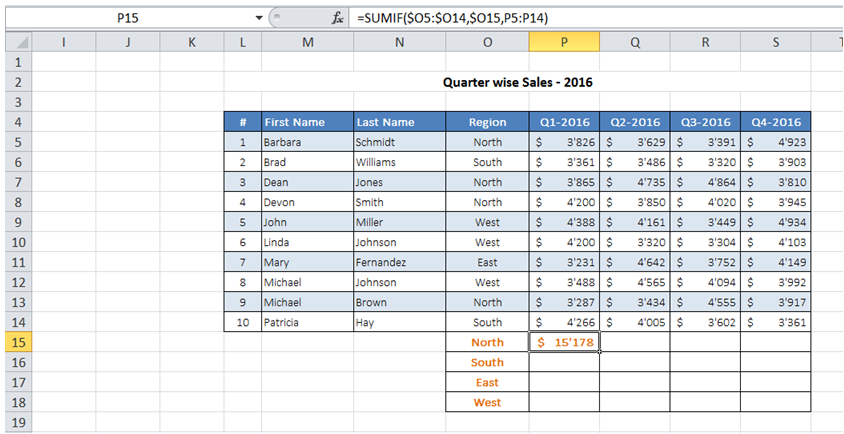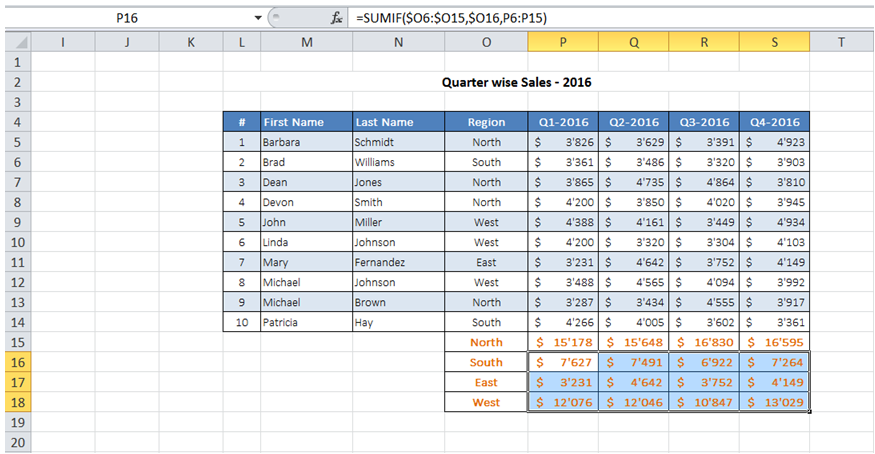## Sum similar record in range in Excel

For example, I want to find the sales contribution in each region from my raw data table.

To do it in Excel, here is the answer:

a) Enter the formula =SUMIF(\$O5:\$O14,\$O15, P5:P14) where O5:O14 has data that corresponds to the criteria, O15 represents the criteria and P5:P15 has sales data that need to be totalled if criteria is met.b) Copy the formula as needed for the data pertaining to other regions and quarters.